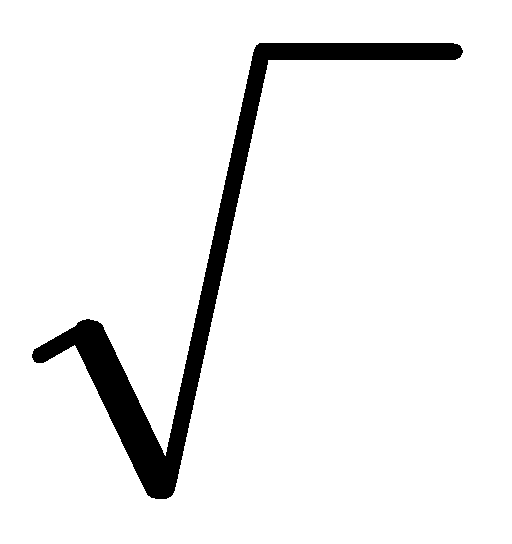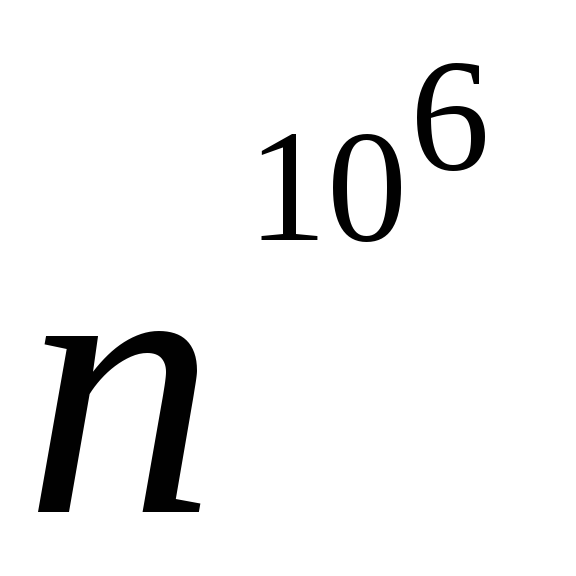# Analysis Of Algorithms University of Bridgeport Analysis of Algorithms

 Page 1/33 Date 28.05.2018 Size 3.4 Mb.

## CS 502Summer 2006

Analysis Of Algorithms University of Bridgeport

Analysis of Algorithms
The field of computer science that studies efficiency of algorithms is known as analysis of algorithms.
Example 1:

Bubblesort requires n2 statements to sort n elements for a given time interval

If computing speed is increased by 1,000,000, then 1,000,000n2 statements can be executed in the same time interval.

But, 1,000,000n2 = (1000n)2

i.e. 1000n elements can be sorted which is anincrease (we lost 3 of 6 orders of magnitude)

Example 2:

Swap down in heapsort requires lg(n) statements for n elements for a given time interval

If computing speed increased by 16, then 16lg(n) statements can be executed in the same time interval.

But, 16lgn = lg(n16) [since]

i.e. n16 elements can be processed, which is an exponential increase

Algorithms for which problem size, which can be solved in the same time interval increase linearly with computing speed are called linear complexity algorithms.

This information has been summarized in the table below:

Table 1: Effect of algorithm efficiency on ability to benefit from increases in speed
 Algorithm Complexity Linear i.e. n Quadratic i.e. n2 Logarithmic i.e. lg(n) Increase in computing speed 1,000,000 (106) 1,000,000 (106) 1,000,000 (106) Equivalent problem size that can be solved in the same amount of time 1,000,000n (106) 1000n (103)(to the power of 106) Benefit from increases in speed LinearExponential

Complexity of Algorithm Depends On Algorithm Implementation:

Example 1: Bubblesort

Code: for i:= 1 to n-1 -----line 1

for j:= 1 to n-1 -----line 2